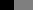# Cel Shading using a ramp/reference texture

I’m looking to make a shader that lights objects using a reference position on a texture based on the value of the light.

I want to take the value of the the light then apply this to a texture containing blocks of black-to-white,so I can edit the threshold for the lighting by just changing the positions of the black/grey/white along the x axis of the lookup texture to create “bands” of light around the object instead of the usual gradient.Currently I have the following code:

``````Shader "Character/Banded Light Ramp Tex" {
Properties {
_MainTex ("Texture", 2D) = "white" {}
_RampTex ("Ramp", 2D) = "white"{}
_Color ("Color", Color) = (1,1,1,1)
_LightCutoff("Maximum distance", Float) = 5.0
}
Tags { "RenderType" = "Opaque" }
CGPROGRAM
#pragma surface surf WrapLambert

uniform float _LightCutoff;
sampler2D _RampTex;

half4 LightingWrapLambert (SurfaceOutput s, half3 lightDir, half atten) {
half NdotL = dot (s.Normal, lightDir);
//atten = step(_LightCutoff, atten) * atten;
atten = atten * NdotL;

// Find position to lookup in ramp texture
float2 lookUpPos = (0.5,saturate(atten));
// Lookup value at position in the ramp texture
atten = (tex2D(_RampTex, lookUpPos));

atten = atten < 1 - _LightCutoff ? 0 : atten;

if(atten <= 0.5)
{
atten = 0;
}

half vMax = (max(max(s.Albedo.r, s.Albedo.g), s.Albedo.b));
half3 colorAdjust = vMax > 0 ? s.Albedo / vMax : 1;
half4 c;
//			if(s.Normal.z > 0 && s.Normal.z < 0.15){
//				atten = 0.5;
//			}
//c.rgb = _LightColor0.rgb * atten;
c.rgb = _LightColor0.rgb * atten * colorAdjust;
c.a = s.Alpha;
return c;
}

struct Input {
float2 uv_MainTex;
float3 viewDir;
};

sampler2D _MainTex;
half4 _Color;
void surf (Input IN, inout SurfaceOutput o) {
o.Albedo = tex2D (_MainTex, IN.uv_MainTex).rgb * _Color;

}
ENDCG
}
Fallback "Diffuse"
}
``````

This code seems to work, but I end up with the light showing as a square under a point light.

I’m not sure if I’m using tex2D incorrectly here. The problem seems to be somewhere in the way I’m looking up the position on the ramp texture. This is my first foray into shaders so I may be missing something incredibly obvious.

EDIT:
Updated code to represent re-written shader. I had to clamp the ramp texture, and change the filter settings in “reimport” and the shader now works mostly as I would like it to. The shader still gives me the weird square shape, which seems to be related to screen size/the facing of the object relative to the camera. I still don’t know why the square is there.

EDIT 2:
Figured out what was causing the square! Atten would be equal to the lowest value in the reference ramp texture if the dot of the normal and the light was zero. The atten should be equal to zero in this case. This caused the shader to use the wrong atten value and draw the strange square.

Figured it all out! See edits on main question for more info. Final code is as follows:

``````Shader "Character/Banded Light Ramp Tex" {
Properties {
_MainTex ("Texture", 2D) = "white" {}
_RampTex ("Ramp", 2D) = "white"{}
_Color ("Color", Color) = (1,1,1,1)
_LightCutoff("Maximum distance", Float) = 5.0
}
Tags { "RenderType" = "Opaque" }
CGPROGRAM
#pragma surface surf WrapLambert

uniform float _LightCutoff;
sampler2D _RampTex;

half4 LightingWrapLambert (SurfaceOutput s, half3 lightDir, half atten) {
half NdotL = dot (s.Normal, lightDir);
atten = atten * NdotL;

// Find position to lookup in ramp texture
float2 lookUpPos = (saturate(atten),saturate(atten));
// Lookup value at position in the ramp texture
atten = (tex2D(_RampTex, lookUpPos));

// Get the lowest value in the ramp texture
float lowVal = tex2D(_RampTex, float2(0,0));
// Check to see if the attenuation is less than the lowest value in the
// ramp texture
if(atten < lowVal)
{
atten = 0;
}

half vMax = (max(max(s.Albedo.r, s.Albedo.g), s.Albedo.b));
half3 colorAdjust = vMax > 0 ? s.Albedo / vMax : 1;
half4 c;
c.rgb = _LightColor0.rgb * atten * colorAdjust;
c.a = s.Alpha;
return c;
}

struct Input {
float2 uv_MainTex;
float3 viewDir;
};

sampler2D _MainTex;
half4 _Color;
void surf (Input IN, inout SurfaceOutput o) {
o.Albedo = tex2D (_MainTex, IN.uv_MainTex).rgb * _Color;

}
ENDCG
}
Fallback "Diffuse"
}
``````